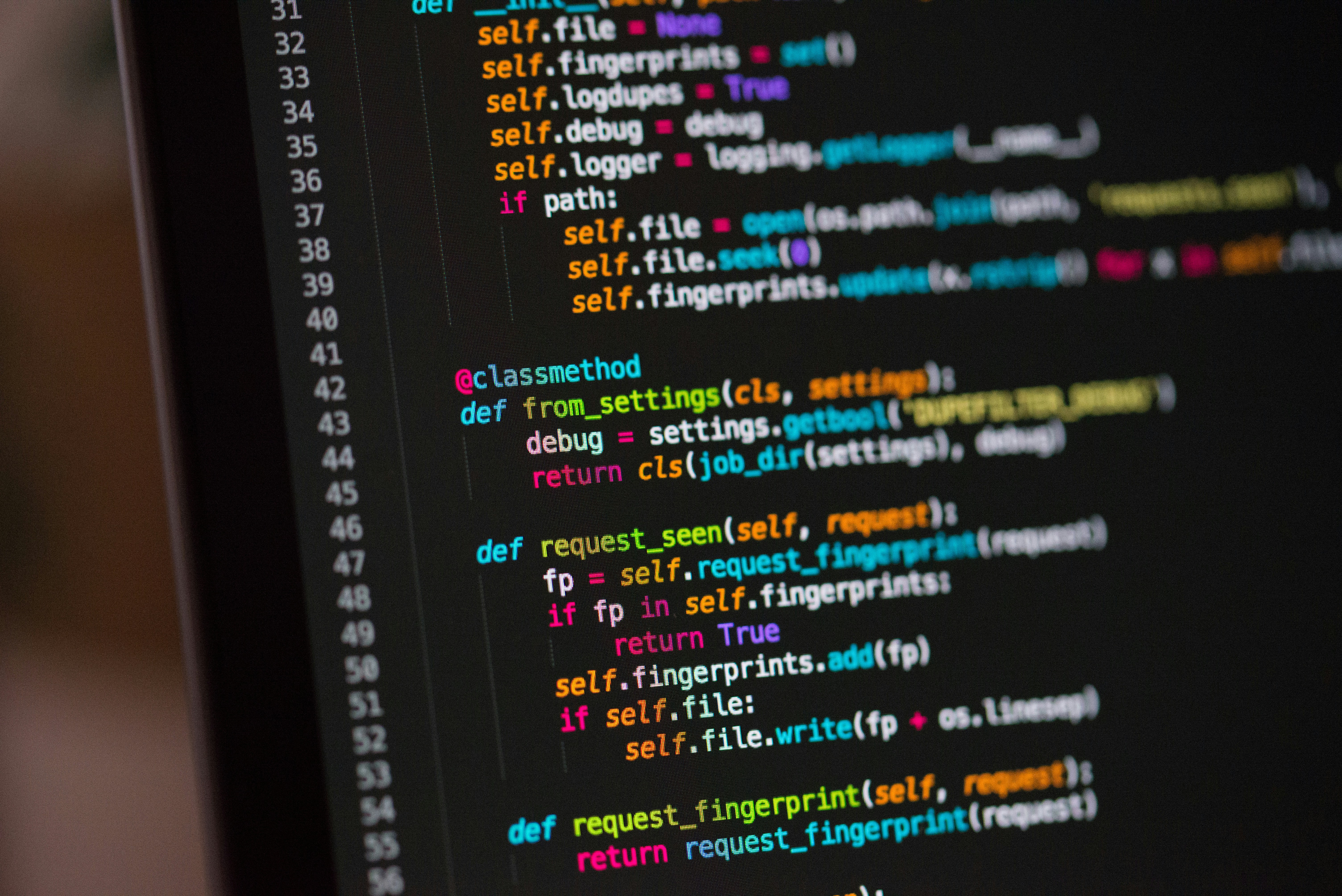Hackerrank Partial Applications Solution

# Hackerrank Partial Applications SolutionIn Partial Application, we create a lambda that takes a parameter and returns a lambda that does something with it.

Example:

multiply_function = -> (number) do
-> (another_number) do
number * another_number
end
end

doubler = multiply_function.(2)
tripler = multiply_function.(3)

puts doubler.(4)
puts tripler.(4)


In the above example, the lambda will take number as a parameter, and return a lambda. When you call this lambda with another_number, it will return the product of the two.

You are given a partially complete code. Your task is to fill in the blanks (_______).

Here, combination is a variable that stores a partial application which computes combination .

### Solution in ruby

combination = -> (n) do
-> (r) do
(n-r+1..n).inject(:*) / (1..r).inject(:*)
end
end

n = gets.to_i
r = gets.to_i
nCr = combination.(n)
puts nCr.(r)